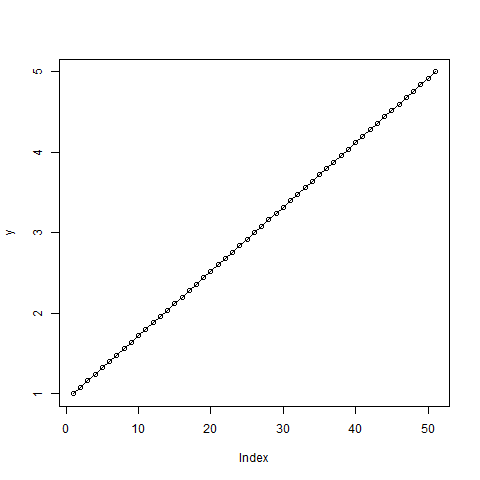# Compute the Value of Quantile Function over Uniform Distribution in R Programming – qunif() Function

• Last Updated : 03 Jul, 2020

In R programming, `qunif()` function gives the value of quantile function over Uniform Distribution.

Syntax:
qunif(p, min, max, lower.tail = TRUE, log.p = FALSE)

Parameters:
p: represents probabilities vector
min, max: represents lower and upper limits of the distribution
lower.tail: represents logical value. If TRUE, probabilities are P[Xx]
log.p: represents logical value. If TRUE, probabilities are given as log(p)

Example 1:

 `# R program to find the value of``# Quantile Function`` ` `# Creating a vector``x <- ``seq``(0, 1, by = 0.2)`` ` `# qunif() function``qunif``(x, min = 2, max = 6)`

Output:

``` 2.0 2.8 3.6 4.4 5.2 6.0
```

Example 2:

 `# Create variables``x <- ``seq``(0, 1, by = 0.02)``y <- ``qunif``(x, min = 1, max = 5)`` ` `# Output to be present as PNG file``png``(file = ``"qunifGFG.png"``)`` ` `# Plot``plot``(y, type = ``"o"``) `` ` `# Saving the file``dev.off``()`

Output:My Personal Notes arrow_drop_up